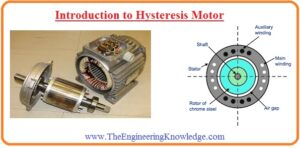Hello, friends welcome to the new post. In this post, we will have a detailed look at Introduction to Hysteresis Motor. The hysteresis motor is the type of synchronous motor that do not need any separate excitation sources like a synchronous motor. It can be function at a single as well as three-phase power source. The torque generation in this motor caused due to the revolving flux induced in the rotor through a flux of the stator.

In this post we will discuss its working structure and some other parameters. So let’s get started with Introduction to Hysteresis Motor.

## Introduction to Hysteresis Motor

• Hysteresis motor belongs to the family of special-purpose motor that uses hysteresis process to generates torque.
• The rotor structure of this motor is the cylindrical shape created with a magnetic substance with the absence of teeth, windings,.
• The stator of can be designed to operate for single and three-phase if it is single phase designed then there is a need of a permanent capacitor to linked with the auxiliary windings. In a result, it generates a smooth field that caused to reduce motor losses.
• The below figure indicate the working of the hysteresis motor.• If three or single phase is connected to the motor current is given to the stator of the motor rotating field exist in the motor.
• This field do magnetization of the metallic portion of rotor and creates magnet poles.
• If the motor rotating speed is less than synchronous speed then 2 sources of torque exist in it.
• Most part of the torque is generated due to the hysteresis. In case the field of stator touches the surface of rotor then rotor flux not follow this path since the metallic part of rotor has larger hysteresis losses.
• With the larger value of intrinsic hysteresis losses at the rotor the larger the angle through which rotor field lags behinds the stator field.
• As the rotor and stator field have different angles than the finite value of torque induced in the motor.
• With that stator field will generate eddy currents in the rotor and these eddy current generates a field in results and that further rises he torque in the rotor.
• The larger the relative speed among the rotor and stator field the larger eddy current and eddy current torques.
• When the motor speed becomes the synchronous speed the stator flux stops to toches the rotor and rotor behaves like a permanent magnet.
• The torque induced in the motor is proportional relation to the angle among the rotor and stator field to the extreme angle adjust by the hysteresis in the rotor.
• The torque-speed characteristic of the hysteresis motor can see n here.• As the quantity of hysteresis in a certain rotor is a function of stator flux density and the material through which it is created. The hysteresis torque of motor is the almost constant value at any speed through 0 to synchronous speed.
• The eddy current torque almost has proportional relation to the slip of the motor.
• these 2 factors apply in sum for the shape of hysteresis motor torque-speed characteristic.
• As the torque of the hysteresis motor for synchronous speed is larger than its extreme synchronous torque the hysteresis motor can rotate at any load which it can operate at normal function.
• The very less hysteresis motor can be designed through shaded pole stator structure configuration to design a less self-starting low power synchronous motor/
• As a motor, that is shown in the below figure.• It is generally used as the driving phenomena in electric clockes. The electrical clock is so synchronized to the line frequency of the power network and the resultant clock is as appropriate as the frequency of the power system that is linked to it.

## Applications of Hysteresis Motor

• The main applications of hysteresis motor are explained here/
• It used in the electrical clock.
• It used in reading players.
• Used in teleprinters
• It used in time-related devices.